Note: This is a working paper which will be expanded/updated frequently. All suggestions for improvement are welcome. The directory deleeuwpdx.net/pubfolders/below has a pdf version, the complete Rmd file with all code chunks, the bib file, and the R source code.

# 1 Introduction

In this paper we study the minimization of stress, defined as $\mathbf{stress}(X):=\frac14\sum_{i=1}^n\sum_{j=1}^n w_{ij}(\delta_{ij}-d_{ij}(X))^2$ over $$n\times p$$ configuration matrices $$X$$. Here $$W=\{w_{ij}\}$$ and $$\Delta=\{\delta_{ij}\}$$ are given symmetric, hollow, and non-negative matricesof, respectively, weights and dissimilarities. The $$d_{ij}(X)$$ are Euclidean distances between the points with coordinates in the rows of $$X$$. Thus $d_{ij}^2(X):=(x_i-x_j)'(x_i-x_j)=(e_i-e_j)'XX'(e_i-e_j)=\mathbf{tr}\ X'A_{ij}X.$ Here $$e_i$$ and $$e_j$$ are unit vectors, with all elements equal to zero except for the single element $$i$$ or $$j$$, which is equal to one. Also $$A_{ij}:=(e_i-e_j)(e_i-e_j)'$$.

The problem of minimizing stress is well-studied (see, for example, Borg and Groenen (2005)) . In this paper we analyze the problem of minimizing stress over all configurations $$X$$ for which $$d_{ij}(X)\leq\alpha_{ij}$$ for some or all pairs $$i,j$$, where the $$\alpha_{ij}$$ are positive bounds. As a special case we have MDS from below, where we require $$d_{ij}(X)\leq\delta_{ij}$$ for all $$i,j$$.

Note that constraints of this type are not exactly independent. If we require $$d_{ij}(X)\leq\alpha_{ij}$$ and $$d_{ik}(X)\leq\alpha_{ik}$$ then, by the triangle inequality, we automatically require $$d_{jk}(X)\leq\alpha_{ij}+\alpha_{ik}$$. On the other hand the constraints are always consistent, because they will be satisfied if we take $$X$$ small enough.

The constraints $$d_{ij}(X)\leq\alpha_{ij}$$, or equivalently $$d_{ij}^2(X)\leq\alpha_{ij}^2$$, define a convex set in configuration space, but stress is not convex. We can, however, use basic smacof theory (De Leeuw (1977)) to majorize stress by a convex quadratic. This can be used to define a simple iterative algorithm. In each iteration we majorize stress, and then minimize the convex quadratic majorizer over configurations satisfying the convex quadratic constraints $$d_{ij}^2(X)\leq\alpha_{ij}^2$$. Compute a new majorization at the minimizer of the majorized problem, and so on. These are the two steps in the majorization or MM approach to optimization (De Leeuw (1994), Lange (2016)). To minimize a convex quadratic under convex quadratic constraints we use the dual Newton method from De Leeuw (2017).

## 1.1 Simplifying stress

A simplified expression for stress, first used in De Leeuw (1993), is $\mathbf{stress}(x)=\frac12\sum_{k=1}^K w_k(\delta_k-\sqrt{x'A_kx})^2.$ We have put the elements below the diagonal of $$W$$ and $$\Delta$$ in a vector of length $$\frac12 n(n-1)$$. Also $$x:=\mathbf{vec}(X)$$ and the $$A_k$$ are the direct sums of $$p$$ copies of the corresponding $$A_{ij}$$. Now expand the square, and assume without loss of generality that $$\frac12\sum_{k=1}^K w_k\delta_k^2=1$$.

Then $\mathbf{stress}(x)=1-\sum_{k=1}^K w_k\delta_k\sqrt{x'A_kx}+\frac12 x'Vx,$ with $$V:=\sum_{k=1}^K w_kA_k$$.

Suppose $$V=K\Lambda^2K'$$ is a complete eigen-decomposition of $$V$$. Some care is needed to handle the fact that $$V$$ is singular, but under the assumption of irreducibility (De Leeuw (1977)) this is easily taken care of. Change variables with $$z=\Lambda^{\frac12}K'x$$. Then $\mathbf{stress}(z)=1-\sum_{k=1}^K w_k\delta_k\sqrt{z'\tilde A_kz}+\frac12 z'z,$ with $$\tilde A_k=\Lambda^{-\frac12}K'A_kK\Lambda^{-\frac12}$$, so that $$\sum_{k=1}^K w_k\tilde A_k=I$$.

## 1.2 Smacof Notation and Theory

We have see in the previous section that we can write $\mathbf{stress}(x)=1-\rho(x)+\frac12 x'x,$ where $\rho(x):=\sum_{k=1}^K w_k\delta_k d_k(x),$ with distances $$d_k(x):=\sqrt{x'A_kx}$$, and the $$A_k$$ positive semi-definite matrices that add up to the identity. By Cauchy-Schwarz, $\rho(x)=x'B(x)x\geq x'B(y)y,$ where $B(x):=\sum_{k=1}^K w_k\frac{\delta_k}{d_k(x)}A_k.$ If we define the Guttman transform if $$x$$ as $$\Gamma(x):=B(x)x$$, then for all $$x$$ $\mathbf(stress)(x)=1-x'\Gamma(x)+\frac12 x'x=(1-\frac12\Gamma(x)'\Gamma(x))+\frac12(x-\Gamma(x))'(x-\Gamma(x)),$ and for all $$x,y$$ $\mathbf(stress)\leq 1-x'\Gamma(y)+\frac12 x'x=(1-\frac12\Gamma(y)'\Gamma(y))+\frac12(x-\Gamma(y)'(x-\Gamma(y)),$ In this notation a smacof iteration is $$x^{(k+1)}=\Gamma(x^{(k)})$$.

## 1.3 Necessary Conditions

We look at the necessary conditions for an optimum of the bounded MDS problem. The Lagrangian is $\mathcal{L}(x,\lambda):=\mathbf{stress}(x)+\frac12\sum_{k=1}^K\lambda_k(x'A_kx-\delta_k^2)$ If $$x$$ is feasible and optimal for the problem of minimizing stress from below then there is a $$\lambda\geq 0$$, not all zero, such that $\mathcal{D}_1\mathcal{L}(x,\lambda)=\left(I+\sum_{k=1}^K\lambda_kA_k\right)x-\Gamma(x)=0$ and the multipliers $$\lambda$$ and constraint functions are complementary, i.e. $$\lambda_k(x'A_kx-\delta_k^2)=0$$ for all $$k$$. Compare this with the necessary condition for a local minimum in regular smacof, which is simply $$x=\\Gamma(x)$$.

It should be noted that $$x=\Gamma(x)$$ implies $$\rho(x)=x'x$$, and consequently at a stationary point $$\sigma(x)=1-\frac12 x'x$$, which implies $$x'x\leq 2$$. Thus $$\sum_{k=1}^K w_kd_k^2(x)\leq 2$$, which implies $$d_k(x)\leq\sqrt{2/w_k}$$. Even in regular smacof, without bound constraints, the distances at a stationary point are bounded, and imposing the constraints $$x'A_kx\leq 2/w_k$$ still allows the unconstrained smacof solution. If the minimum stress is non-zero, then none of the constraints will be active, and all the distances will be strictly smaller than the a priori bounds.

# 2 MDS from Below

Classical MDS (Torgerson (1958)) can be interpreted as minimizing the loss function strain, defined as $\mathbf{strain}(X):=\frac14\mathbf{tr}\ J_n(\Delta^2-D^2(X))J_n(\Delta^2-D^2(X)),$ over all $$n\times p$$ centered configuration matrices $$X$$, where $$J_n$$ is the centering operator $$J_n=I_n-\frac{1}{n}ee'$$, $$\Delta^2$$ is a matrix with squared dissimilarities, and $$D^2(X)$$ is a matrix of squared Euclidean distances.

The loss function interpretation is based on the identity $-\frac12 J_nD^2(X)J_n=XX',$ which implies $\mathbf{strain}(X)=\mathbf{tr}\ (C-XX')^2,$ with $$C:=-\frac12J_n\Delta^2J_n$$.

If $$C$$ has signature $$(n_+,n_0,n_-)$$ then we can write $$C=\overline{C}-\underline{C}$$ with both $$\overline{C}$$ and $$\underline{C}$$ positive semi-definite, of ranks $$n_+$$ and $$n_-$$, and with $$\mathbf{tr}\ \overline{C}\underline{C}=0$$. $$\overline{C}$$ is the projection of $$C$$ on the convex cone of positive semi-definite matrices, and $$\underline{C}=-(C-\overline{C})$$ is the negative of the projection on its polar cone. In terms of the eigenvalues and eigenvectors of $$C$$ we can write $$C=\overline{K}\overline{\Lambda}\overline{K}'+\underline{K}\underline{\Lambda}\underline{K}$$, with overlining used for the positive eigenvalues and their eigenvectors, and underlining for the negative ones. Thus $\mathbf{strain}(X)=\mathbf{tr}\ (\overline{C}-\underline{C}-XX')^2=\mathbf{tr}\ (\overline{C}-XX')^2+\mathbf{tr}\ \underline{C}^2+2\mathbf{tr}\ X'\underline{C}X,$ and $\min_X\mathbf{strain}(X)\geq\min_Z\mathbf{tr}\ (\overline{C}-ZZ')^2+\mathbf{tr}\ \underline{C}^2+2\min_Y\mathbf{tr}\ Y'\underline{C}Y=\min_Z\mathbf{tr}\ (\overline{C}-ZZ')^2+\mathbf{tr}\ \underline{C}^2.$ Thus if $$p\geq n_+$$ we choose $$Z$$ so that $$ZZ'=\overline{C}$$, if $$p<n_+$$ we use the $$p$$ largest eigenvalues and corresponding eigenvectors of $$\overline{C}$$ to find $$ZZ'$$.

Now consider the squared distances $$d_{ij}^2(\overline{C})=\overline{c}_{ii}+\overline{c}_{jj}-2\overline{c}_{ij}$$ and the squared distances $$d_{ij}^2(X_r)=\sum_{s=1}^r(x_{is}-x_{js})^2$$. Then for all $$i,j$$ $d_{ij}^2(X_1)\leq d_{ij}^2(X_2)\leq\cdots\leq d_{ij}^2(X_{n+})=d_{ij}^2(\overline{C}).$ Thus solutions are nested and we use quadratic approximation from below of the positive semi-definite part of $$C$$ (De Leeuw and Meulman (1986))

The idea of approximation from below, which is natural in the case of classical MDS, can also be applied to the minimization of stress, using the coinstraints $$d_{ij}(X)\leq\delta_{ij}$$ for all $$i$$ and $$j$$.

This may seem somewhat perverse, because why would we ever impose such constraints ? In this context, however, it makes sense to interpret the $$\delta_{ij}$$ as bounds instead of dissimilarities. The problem is to locate objects in space in such a way that their distances do not exceed their upper bounds, and are as close to these bounds as possible. Thus the upper bound problem is perhaps more like a location problem than a multidimensional scaling problem.

# 3 Examples

## 3.1 Equidistances

Our first example has $$n=10$$ with all $$\delta_{ij}$$ equal. The optimal smacof solution in two dimensions needs 131 iterations to arrive at stress 0.1098799783. Approximation from below uses 30 iterations and finds stress 0.1520077612. The two configurations are in figure 1. Note they produce two different local minima, one with nine points equally spaced on the circle and a tenth point in the center, and the other with ten points equally spaced on the circle. At the solution of the bounded problem there are 10 active constraints, which have $$d_{ij}(X)=\delta_{ij}(X)=1$$.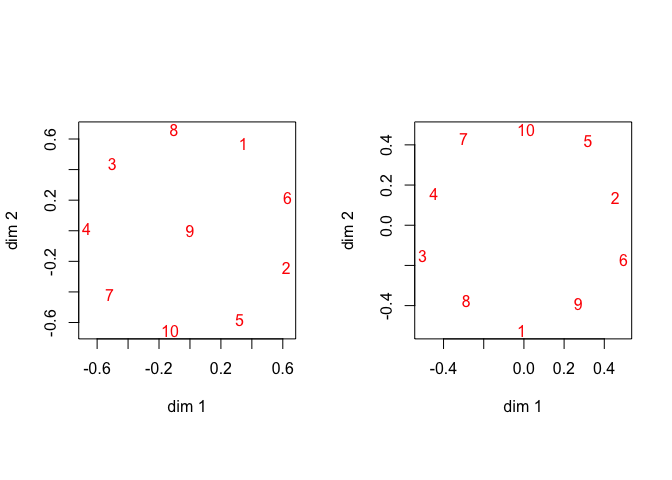Figure 1: Equidistance Data, Regular Left, From Below Right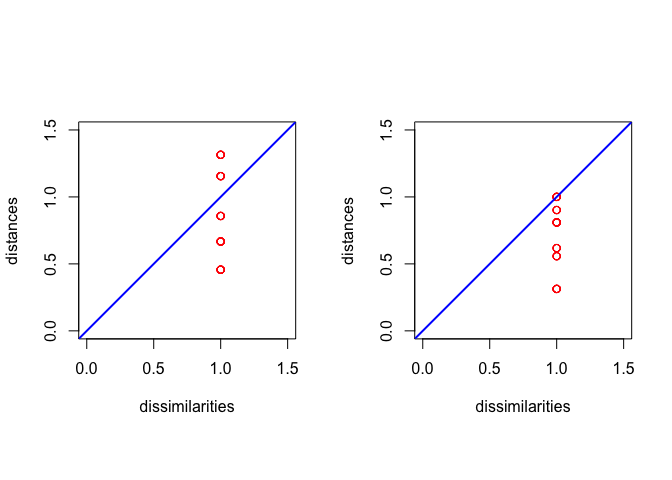Figure 2: Equidistance Data, Regular Left, From Below Right

To illustrate complementary slackness we give the values of the constraint functions $$d_{ij}^2(X)-\delta_{ij}^2$$, which are all non-positive, and the values of the Lagrange multipliers, which are all non-negative. We see that a constraint function that is equal to zero means a positive Lagrange multiplier, and a constraint function that is negative means a zero Lagrange multiplier.

mprint (h2b$constraints, d = 6, f = "+") ##  -0.172287 -0.309015 -0.172287 +0.000000 -0.309015 +0.000000 -0.451059 ##  -0.451058 +0.000000 +0.000000 -0.093093 -0.451058 -0.451058 -0.172283 ##  -0.093098 -0.344574 -0.344576 -0.451059 +0.000000 +0.000000 -0.309023 ##  -0.451053 -0.172291 -0.172292 -0.172283 +0.000000 -0.451058 -0.344574 ##  -0.093098 -0.344576 -0.309023 -0.309009 +0.000000 -0.172288 -0.451054 ##  +0.000000 -0.172292 -0.451053 -0.172292 -0.172287 +0.000000 -0.451055 ##  -0.344584 -0.093096 -0.093096 mprint (h2b$multipliers, d = 6, f = "+")
##   +0.000000 +0.000000 +0.000000 +0.088502 +0.000000 +0.088503 +0.000000
##   +0.000000 +1.220853 +1.220844 +0.000000 +0.000000 +0.000000 +0.000000
##  +0.000000 +0.000000 +0.000000 +0.000000 +0.088508 +0.088477 +0.000000
##  +0.000000 +0.000000 +0.000000 +0.000000 +1.220844 +0.000000 +0.000000
##  +0.000000 +0.000000 +0.000000 +0.000000 +1.220840 +0.000000 +0.000000
##  +0.088508 +0.000000 +0.000000 +0.000000 +0.000000 +1.220840 +0.000000
##  +0.000000 +0.000000 +0.000000

## 3.2 Dutch Political Parties 1967

As the next illustration we use data from De Gruijter (1967), with average dissimilarity judgments between Dutch political parties in 1967. The data are

##       KVP PvdA  VVD  ARP  CHU  CPN  PSP   BP
## PvdA 5.63
## VVD  5.27 6.72
## ARP  4.60 5.64 5.46
## CHU  4.80 6.22 4.97 3.20
## CPN  7.54 5.12 8.13 7.84 7.80
## PSP  6.73 4.59 7.55 6.73 7.08 4.08
## BP   7.18 7.22 6.90 7.28 6.96 6.34 6.88
## D66  6.17 5.47 4.67 6.13 6.04 7.42 6.36 7.36

The optimal smacof solution in two dimensions needs 319 iterations to arrive at stress 0.044603386. Approximation from below uses 32 iterations and finds stress 0.0752702106. At the solution there are 10 active constraints, but as the Shepard plots in figure 4 show, the active constraints now do not correspond with the largest dissimilarities, and the two configurations in figure 3 are quite different. Although that could, of course, also be because they correspond with two different local minima, which mostly differ in the location of the D66 party (a neoliberal party, full of civilized protest).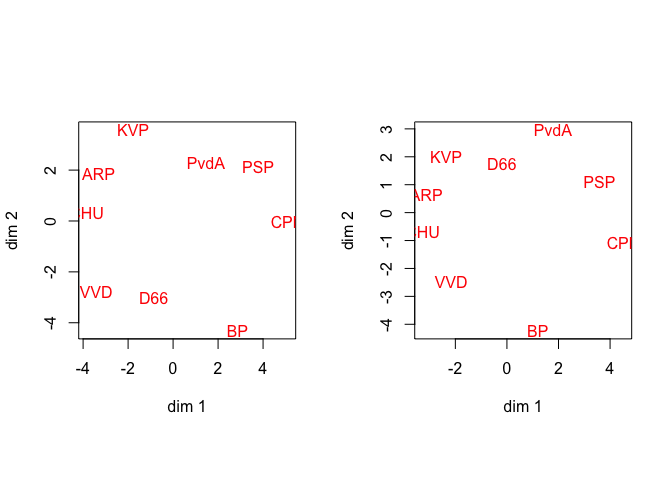Figure 3: De Gruijter Data, Regular Left, From Below Right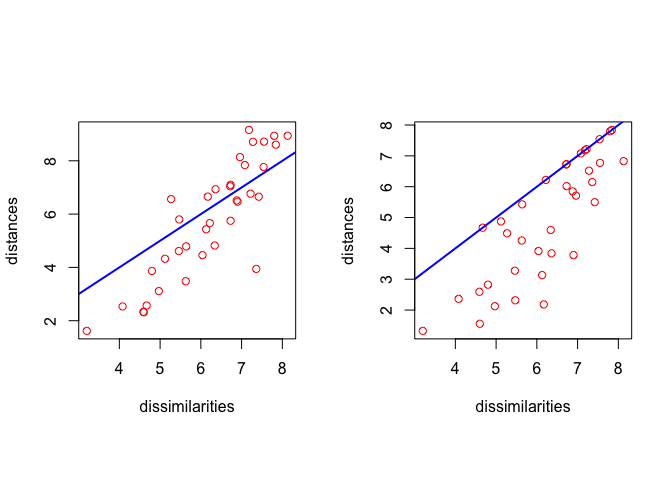Figure 4: De Gruijter Data, Regular Left, From Below Right

In the next analysis we require that all distances are less than or equal to 8.13, the maximum dissimilarity. In order words, the maximum distances must be less than or equal to the maximum dissimilarity.

The optimal solution under these constraints in two dimensions needs 70 iterations to arrive at stress 0.0537645693. At the solution there are 6 active constraints, i.e. six distances equal to 8.13.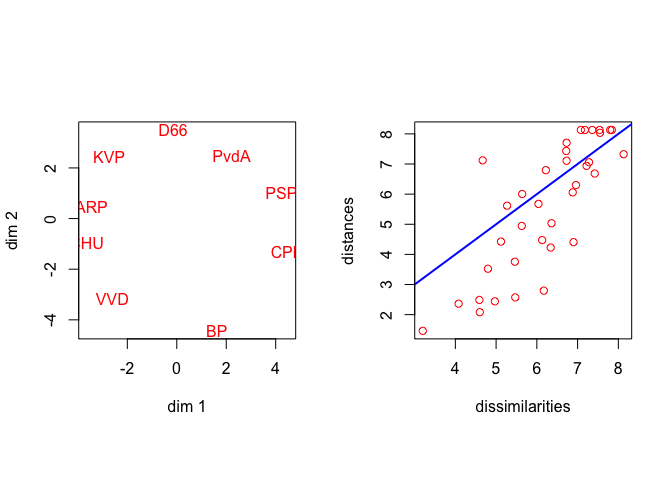Figure 5: De Gruijter Data, Distances Bounded by Maximum Dissimilarity

And finally an analysis in which we require that distances between ARP, CHU, KVP (the Christian Democrat parties) are less than 0.1, and the distances between PvdA, PSP, CPN (the leftist parties) are also less than 0.1. This will effectively collapse them in the configuration plot.

The optimal solution under these constraints in two dimensions needs 196 iterations to arrive at stress 0.0494598844. At the solution all 1 constraints are active.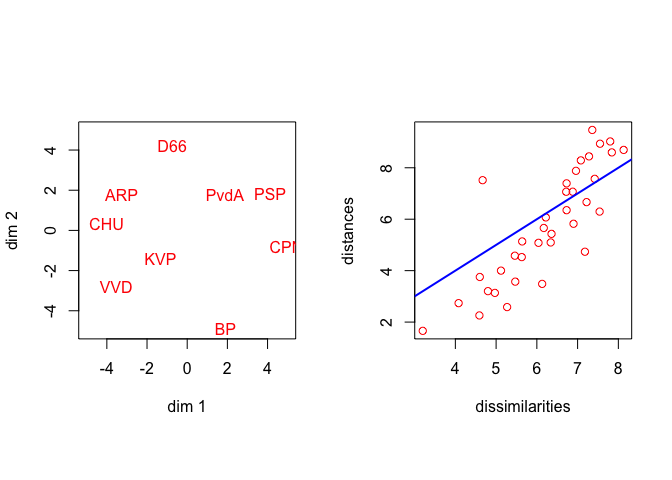Figure 6: De Gruijter Data, Imposing Small Distances

## 3.3 Ekman Color Data

The final example are the color data from Ekman (1954). The optimal smacof solution in two dimensions needs 26 iterations to arrive at stress 0.0172132469. Approximation from below uses 16 iterations and finds stress 0.0274106473. At the solution there are 15 active constraints. Again the bounded solution is a shrunken version of the regular smacof solution, and figure 8 shows that the active constraints correspond with the largest dissimilarities and distances.